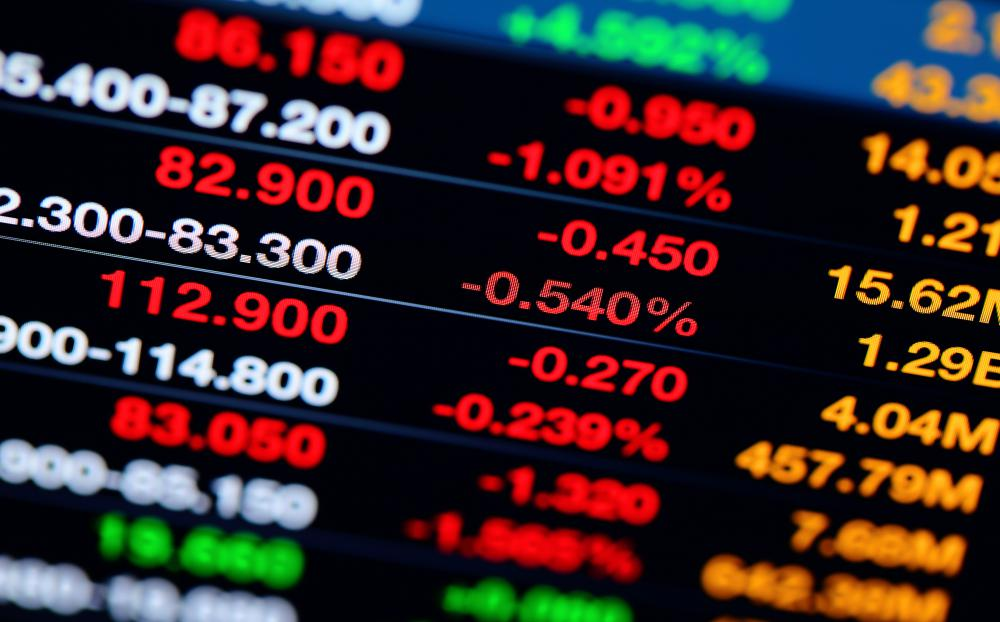# What is a Conversion Parity Price?

Malcolm Tatum

A conversion parity price is the calculation of the eventual realized rate or price that is paid for any type of convertible security that is later converted into shares of common stock. The conversion parity price is not considered to be the same as the actual purchase price for the security. Sometimes referred to as a market conversion price, the conversion parity price seeks to determine how equal the price between the security and that value of the stocks acquired by the conversion process actually turns out to be.A conversion parity price is the price that is paid for any type of convertible security that is later converted into shares of common stock.

Calculating the conversion parity price involves two key pieces of information. It is necessary to know the conversion ratio set for the security at the time of purchase. In addition, the actual purchase price paid for the security must be available. Determining the conversion party price involves dividing the purchase price of the security by the conversion ratio.

If the purpose of calculating the conversion parity price is to determine the realized price involved when converting shares of common stock into a convertible security, then the formula is slightly different but still very simple. With this type of conversion option approach, the process would involve dividing the market value of the shares of common stock by the conversion ratio.Get started

Taking the time to calculate the conversion parity price is one important tool for anyone who wishes to invest in convertible securities. Along with paying close attention to the level of conversion ratio, going through the necessary steps to ascertain the conversion parity price as it currently stands can provide some insight into the potential for future profits from acquiring the security. Because the process for calculating the conversion parity price is straightforward, investors and brokers can assess the viability of making the purchase within minutes. If utilized as a means of evaluating the overall potential related to the investment, figuring the conversion parity price can make the difference in getting in on a great deal or losing a fair amount of money at some future point.

## You might also Like